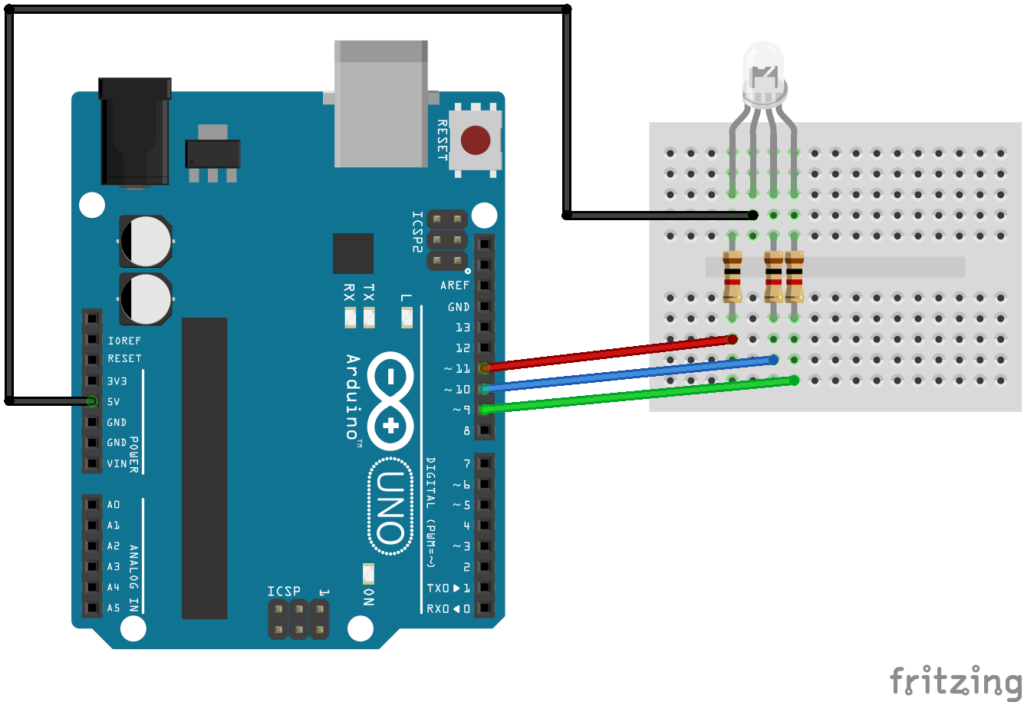Arduino for beginners : do while loop

The do loop works in the same manner as the while loop, with one difference in that the condition is tested at the end of the loop, so the do loop will always run at least once even if the test expression was false.

The do while loop is shown below.

do {
Statement 1
Statement 2

} while (test expression);
Code Examples

OK, here is the standard do while equivalent of the previous for loop and while loop examples

 Source code```void setup()
{
int i = 1;
Serial.begin(9600);
do
{
Serial.print("i = ");
Serial.println(i);
i++;
}while(i<=10);
}
void loop() {
}```

Open the serial monitor window and you will see the following, looks good

i = 1
i = 2
i = 3
i = 4
i = 5
i = 6
i = 7
i = 8
i = 9
i = 10
Just for fun, lets remove the i++ from the code above and run again and look at the serial monitor window

i = 1
i = 1
i = 1
i = 1
i = 1
i = 1
i = 1
i = 1
i = 1
i = 1
i = 1
i = 1
i = 1

As you can see because the varable wasn’t incremented then the test expression will always be true , i will always be less than or equal to 10. Infinite loop.

Back to the RGB led againNow lets repeat the fading an led and flashing an led 10 times examples

 Source code```//change the brightness of an LED
int redled = 11;
int i =1;
void setup()
{
pinMode(redled, OUTPUT); //set the LED pin as an output
Serial.begin(9600);
do{
Serial.print("loop = ");
Serial.println(i);
analogWrite(redled, i);
delay(10);
i++;
}while(i<=255);
}
void loop()
{
}```

Now flash the LED 10 times again

 Source code```//flash the red led of the RGB led 10 times
int redled = 11;
int i = 1; //init the variable
void setup()
{
Serial.begin(9600);
pinMode(redled, OUTPUT); //set the LED pin as an output
//this will run 10 times
do
{
Serial.print("loop = ");
Serial.println(i);
digitalWrite(redled, LOW); // led on
delay(500);
digitalWrite(redled, HIGH); //led off
delay(500);
i++; //dont forget this
}while(i<=10);
}
void loop()
{
}```